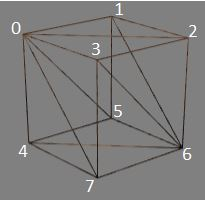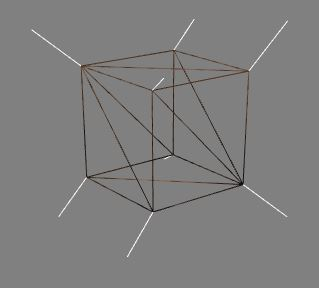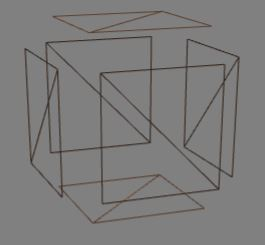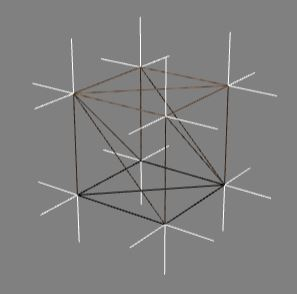# Vertex Normals

## Vertex Normals

Each triangular facet of a mesh comprises three vertices. Besides a position each vertex has another important vector3 called a normal. These vertex normals are used by the shader code in calculating how the mesh is lit. Unlike a mathematical normal there is no necessity for them to be set at right angles and for curved shapes such as a sphere they may not be. In the case of a sphere they are set as the mathematical normal of the sphere surface rather than that of the flat facets of the mesh that create the sphere.

At first the vertex normals are calculated as the mathematical normals for the facet. It then depends whether you want to view the facets as flat surfaces or as part of curve. For flat surfaces the vertex normals remain as the mathematical normals. To enhance the curve when viewed under light where triangular facets share vertices with the same positions each shared vertex normal is recalculated to be the average of the mathematical normals of the shared vertex normals.

These effects are explored below.

In the following two playgrounds see how the changing directions within the normals array affect how it is lit:

Vertex Normals Varying In Unison Showing Normals Varying

##Normals and Minimum VerticesThe box above has 8 vertices. If we want to keep the indices to the minimum they will be 0, 1, 2, 3, 4, 5, 6, 7.

Facets 0, 3, 7 and 3, 7, 6 and 0, 3, 2 all have vertex 3 in common and vertex 3 can only have one entry in the normals array associated with it.

How doesBabylon.js calculate the normal for vertex 3?

The diagram below shows that the average of the three mathematical normals at each vertex is used:Besides minimising the number of vertices needed there are other advantages as will be seen when creating a sphere.

Keeping the indices to a minimum the normals at each corner are an average of the mathematical normals of the three faces that meet at that corner. So 8 corners and 8 unique vertex normals.

## Table of Unique Indices, Positions and Normals for Box with Minimum Vertices

indexpositionnormal
index
0
position
( -1 , 1 , -1 )
normal
( -0.5773502691896258 , 0.5773502691896258 , -0.5773502691896258 )
index
1
position
( 1 , 1 , -1 )
normal
( 0.8164965809277261 , 0.4082482904638631 , -0.4082482904638631 )
index
2
position
( 1 , -1 , -1 )
normal
( 0.4082482904638631 , -0.4082482904638631 , -0.8164965809277261 )
index
3
position
( -1 , -1 , -1 )
normal
( -0.4082482904638631 , -0.8164965809277261 , -0.4082482904638631 )
index
4
position
( -1 , 1 , 1 )
normal
( -0.4082482904638631 , 0.4082482904638631 , 0.8164965809277261 )
index
5
position
( 1 , 1 , 1 )
normal
( 0.4082482904638631 , 0.8164965809277261 , 0.4082482904638631 )
index
6
position
( 1 , -1 , 1 )
normal
( 0.5773502691896258 , -0.5773502691896258 , 0.5773502691896258 )
index
7
position
( -1 , -1 , 1 )
normal
( -0.8164965809277261 , -0.4082482904638631 , 0.4082482904638631 )

## Normals and Flat Shaded Meshes.

There are times, such as needing each face of a box to be covered in a different material, when it is better to have the box constructed from separate faces each of which are constructed by two facets and no two faces sharing a vertex indices. They will of course share vertex positions.In Babylon.js this can be achieved using the convertToFlatShadedMesh function. The results are shown below:For a flat shaded mesh each of the triangular facets making a face of the box has mathematical normals as their vertex normals. For simplicity of illustration we will only consider the the six faces making up the box than the full range of triangular facets used in the mesh construction. Each face has 4 corners, each corner has a unique normal at right angles to the face. There are 6 faces on a box and so 24 unique corner normals.

## Table of Faces, Corners, Positions and Normals for Flat Shaded Box

Facecornerpositionnormal
Face
Front
corner
0
position
( -1 , 1 , -1 )
normal
( 0 , 0 , -1 )
Face
Front
corner
1
position
( 1 , -1 , -1 )
normal
( 0 , 0 , -1 )
Face
Front
corner
2
position
( 1 , 1 , -1 )
normal
( 0 , 0 , -1 )
Face
Front
corner
3
position
( -1 , 1 , -1 )
normal
( 0 , 0 , -1 )
Face
Back
corner
4
position
( -1 , 1 , 1 )
normal
( 0 , 0 , 1 )
Face
Back
corner
5
position
( 1 , -1 , 1 )
normal
( 0 , 0 , 1 )
Face
Back
corner
6
position
( -1 , -1 , 1 )
normal
( 0 , 0 , 1 )
Face
Back
corner
7
position
( -1 , 1 , 1 )
normal
( 0 , 0 , 1 )
Face
Right
corner
8
position
( 1 , 1 , -1 )
normal
( 1 , -0 , 0 )
Face
Right
corner
9
position
( 1 , -1 , 1 )
normal
( 1 , -0 , 0 )
Face
Right
corner
10
position
( 1 , 1 , 1 )
normal
( 1 , -0 , 0 )
Face
Right
corner
11
position
( 1 , 1 , -1 )
normal
( 1 , 0 , 0 )
Face
Left
corner
12
position
( -1 , 1 , -1 )
normal
( -1 , 0 , 0 )
Face
Left
corner
13
position
( -1 , -1 , 1 )
normal
( -1 , 0 , 0 )
Face
Left
corner
14
position
( -1 , -1 , -1 )
normal
( -1 , 0 , 0 )
Face
Left
corner
15
position
( -1 , 1 , -1 )
normal
( -1 , -0 , -0 )
Face
Top
corner
16
position
( -1 , 1 , -1 )
normal
( 0 , 1 , 0 )
Face
Top
corner
17
position
( 1 , 1 , 1 )
normal
( 0 , 1 , 0 )
Face
Top
corner
18
position
( -1 , 1 , 1 )
normal
( 0 , 1 , 0 )
Face
Top
corner
19
position
( 1 , 1 , -1 )
normal
( 0 , 1 , -0 )
Face
Base
corner
20
position
( -1 , -1 , -1 )
normal
( 0 , -1 , -0 )
Face
Base
corner
21
position
( 1 , -1 , 1 )
normal
( 0 , -1 , -0 )
Face
Base
corner
22
position
( 1 , -1 , -1 )
normal
( 0 , -1 , -0 )
Face
Base
corner
23
position
( -1 , -1 , 1 )
normal
( 0 , -1 , 0 )

## Playground Showing Box Normals

Box Normals

Applying the function converToFlatShadedMesh shows the individual faces making up the sphere. For a flat shaded sphere the normals of each facet are the mathematical normals of the facet.